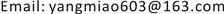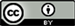﻿ 基于优化GM(1,1)灰色模型预测成都市老年人口 Prediction of Population Aging in Chengdu: A Study Based on Optimized Grey GM(1,1) Model

Statistics and Application
Vol.06 No.04(2017), Article ID:22411,6 pages
10.12677/SA.2017.64045

Prediction of Population Aging in Chengdu: A Study Based on Optimized Grey GM(1,1) Model

Miao Yang

L.A.S Department of CDUESTC, Chengdu College of University of Electronic Science and Technology of China, Chengdu SichuanReceived: Oct. 2nd, 2017; accepted: Oct. 16th, 2017; published: Oct. 23rd, 2017ABSTRACT

On the basis of data of 2009~2016 population aging, the paper uses Optimized Grey GM(1,1) model and metabolic GM(1,1) model, with testing and programming calculation of MATLAB software, to predict population aged 60 and older between 2017~2022 of Chengdu City. Model accuracy test shows that the model accuracy is good. The model prediction results are high reliability and believability. The model is suitable for the long-term prediction of population.

Keywords:Optimized Grey GM(1,1) Model, Population Aging, Metabolic GM(1,1) Model1. 引言

2. 数学模型简介

2.1. GM(1,1)模型

GM(1,1)模型是基于累加生成地数列预测模型，建立地步骤为：

1) ${x}^{\left(0\right)}\left(1\right),{x}^{\left(0\right)}\left(2\right),\cdots ,{x}^{\left(0\right)}\left(n\right)$ 是所要预测地某项指标地原始数据。对原始数据作一次累加生成处理，

${x}^{\left(1\right)}\left(n\right)=\underset{i=1}{\overset{n}{\sum }}{x}^{\left(0\right)}\left(t\right)$ ，得到一个新地数列。这个新的数列与原始数列相比，其随机性程度大大弱化，平

2) 将新数列的变化趋势近似地用微分方程描述， $\frac{\text{d}{x}^{\left(1\right)}}{\text{d}t}+\alpha {x}^{\left(1\right)}=\mu$ ，其中， $\alpha ,\mu$ 为辨识参数。辨识参数通过最小二乘法拟合得到， $\left[\begin{array}{l}\alpha \\ \mu \end{array}\right]={\left({B}^{\text{T}}B\right)}^{-1}{B}^{\text{T}}{Y}_{n}$

3) 构造矩阵。 ${Y}_{n}={\left[{x}^{\left(0\right)}\left(2\right),{x}^{\left(0\right)}\left(3\right),\cdots ,{x}^{\left(0\right)}\left(n\right)\right]}^{\text{T}}$$B$ 为构造数据矩阵

$B=\left[\begin{array}{cc}-\frac{1}{2}\left[{x}^{\left(1\right)}\left(1\right)+{x}^{\left(1\right)}\left(2\right)\right]& 1\\ -\frac{1}{2}\left[{x}^{\left(1\right)}\left(2\right)+{x}^{\left(1\right)}\left(3\right)\right]& 1\\ ⋮& ⋮\\ -\frac{1}{2}\left[{x}^{\left(1\right)}\left(n-1\right)+{x}^{\left(1\right)}\left(n\right)\right]& 1\end{array}\right]$ (1)

4) 求出预测模型

${\stackrel{^}{x}}^{\left(1\right)}\left(t+1\right)=\left[{x}^{\left(0\right)}\left(1\right)-\frac{\mu }{\alpha }\right]{\text{e}}^{-\alpha t}+\frac{\mu }{\alpha }$ . (2)

5) 进行一次数据累减，得到预测方程：

${\stackrel{^}{x}}^{\left(0\right)}\left(t+1\right)={\stackrel{^}{x}}^{\left(1\right)}\left(t+1\right)-{\stackrel{^}{x}}^{\left(1\right)}\left(t\right)$ . (3)

2.2. 优化GM(1,1)模型 

${z}^{\left(1\right)}\left(t\right)=\frac{1}{2}\left({x}^{\left(1\right)}\left(t\right)+{x}^{\left(1\right)}\left(t-1\right)\right)$ 的构造形式。即背景值的构造公式是导致模拟误差及GM(1,1)模型的适应

${z}^{\left(1\right)}\left(t\right)={\int }_{t-1}^{t}{x}^{\left(1\right)}\left(t\right)\text{d}t$ . (4)

${x}^{\left(1\right)}\left(t\right)=B{\text{e}}^{At}$ ，其中 $A,B$ 为待定常数，且满足 ${x}^{\left(1\right)}\left(t\right)=B{\text{e}}^{At},\left(t=1,2,\cdots ,n\right)$ ，将 ${x}^{\left(1\right)}\left(t\right)=B{\text{e}}^{At}$ 代入(4)得

${z}^{\left(1\right)}\left(t\right)={\int }_{\begin{array}{c}t-1\end{array}}^{t}B{\text{e}}^{At}\text{d}t=\frac{1}{A}\left[B{\text{e}}^{At}-B{\text{e}}^{A\left(t-1\right)}\right]=\frac{1}{A}\left[{x}^{\left(1\right)}\left(t\right)-{x}^{\left(1\right)}\left(t-1\right)\right]$ (5)

$A=\mathrm{ln}{x}^{\left(1\right)}\left(t\right)-\mathrm{ln}{x}^{\left(1\right)}\left(t-1\right)$ . (6)

${z}^{\left(1\right)}\left(t\right)=\frac{{x}^{\left(1\right)}\left(t\right)-{x}^{\left(1\right)}\left(t-1\right)}{\mathrm{ln}{x}^{\left(1\right)}\left(t\right)-\mathrm{ln}{x}^{\left(1\right)}\left(t-1\right)},k=2,3,\cdots ,n$ (7)

2.3. 新陈代谢GM(1,1)模型 

3. 实例分析

3.1. 预测模型的建立

${B}^{\text{T}}=\left[\begin{array}{ccccccc}-256.6& -499.4& -719.8& -950.1& -1193.2& -1448.1& -1727.7\\ 1& 1& 1& 1& 1& 1& 1\end{array}\right]$

${Y}_{n}=\left[400,614,837,1073,1322,1582,1882\right]$

$\left\{\begin{array}{l}{\stackrel{^}{x}}^{\left(1\right)}\left(t+1\right)=3129.1{\text{e}}^{0.0616t}-2932.1\\ {\stackrel{^}{x}}^{\text{0}}\left(t+1\right)={\stackrel{^}{x}}^{\left(1\right)}\left(t+1\right)-{\stackrel{^}{x}}^{\left(1\right)}\left(t\right)\end{array}$

3.2. 模型检验

1) 残差检验。

2) 关联度检验。

${\Delta }_{i}=\left[{x}_{i}^{\left(l\right)}\left(t\right)-{x}_{i}^{\left(0\right)}\left(t\right)\right]$ ，通过关联度检验的标准就是关联系数大于0.6，当关联度检验通过时，我们给出的预测模型便是满意。

3) 后验差检验。检验残差分布的统计特性   。Table 1. The number of population aging in Chengdu (unit: ten thousand people)

${S}_{1}=\sqrt{\frac{\sum {\left({x}^{\left(0\right)}\left(t\right)-{\stackrel{^}{x}}^{\left(0\right)}\left(t\right)\right)}^{2}}{n-1}}$

${S}_{2}=\sqrt{\frac{\sum {\left({\Delta }^{\left(0\right)}\left(t\right)-{\stackrel{^}{\Delta }}^{\left(0\right)}\left(t\right)\right)}^{2}}{n-1}}$

3.3. 成都市老年人口预测Table 2. The grade standard of model predictive accuracy testTable 3. The actual value of the population aging in Chengdu in 2011~2016: Comparison of general model and optimization modelTable 4. The prediction value of population aging in Chengdu in 2017-2022 (unit: ten thousand people)

4. 结束语

Prediction of Population Aging in Chengdu: A Study Based on Optimized Grey GM(1,1) Model[J]. 统计学与应用, 2017, 06(04): 396-401. http://dx.doi.org/10.12677/SA.2017.64045

1. 1. 朱伟. 灰色预测模型及其应用[J]. 数学杂志, 1998, 18: 133-135.

2. 2. 刘思峰, 党耀国, 方志耕, 等. 灰色系统理论及其应用[M]. 北京: 科学出版社, 2010.

3. 3. 周瑞平. GM(1,1)模型灰色预测法预测城市人口规模[J]. 内蒙古师范大学学报, 2005, 34(1): 81-83.

4. 4. 罗党, 刘思峰, 党耀国. 灰色模型GM(1,1)优化[J]. 中国工程科学, 2003, 5(8): 50-53.

5. 5. 王宁, 张爽, 曾庆均. 基于新陈代谢GM(1,1)模型的重庆市人口老龄化预测研究[J]. 西北人口, 2017, 1(38): 66-70.

6. 6. 王宁, 张爽. 基于GM(1,1)模型的重庆市五大功能区人口老龄化预测[J]. 中国商论, 2016(20): 170-171.

7. 7. 金浩, 张珺, 王志双. 基于组合预测的公路货运量需求预测实证研究——以唐山市为例[J]. 统计与管理, 2016(1): 52-55.